# Civil Engineering - UPSC Civil Service Exam Questions

6.

A bar AB, 4 cm is diameter and 4 m long is rigidly fixed at its ends. A torque of 12000 kg/cm is applied at a section of the bar, one metre from end A. The fixing coupled TA and TB (in kg. cm) at the supports A and B will be respectively

 A. 9000, 6000 B. 3000, 9000 C. 6000, 9000 D. 9000, 3000

Explanation:

No answer description available for this question. Let us discuss.

7.

Consider the following assumptions of Bowditch method :
1. Angular measurements are more precise than linear measurements.
2. Linear measurements are more prescise than angular measurements.
3. Erros in linear measurements are proportional to L.
4. Correction to latitude or departure of any side = Total error in L (or D) x Length of that side / Perimeter of traverse.
Which of these statements are correct ?

 A. 1 and 4 B. 1, 2 and 3 C. 2, 3 and 4 D. 3 and 4

Explanation:

No answer description available for this question. Let us discuss.

8.

For the beam shown in the given figure, the collapse load P is given by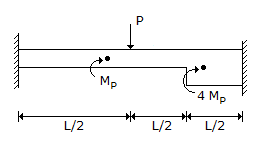A.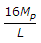B.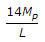C.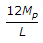D.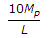Explanation:

No answer description available for this question. Let us discuss.

9.

For a laminated spring, l = length, n = number of plates, width of each plate = b, thickness of each plate = t, central point load = W, what is the expression for the maximum bending stores ?

 A.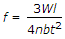* 2 B.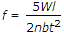C.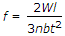D.Explanation:

No answer description available for this question. Let us discuss.

10.

Two particles of masses 21 gm and 28 gm are connected by a light inextensible string which passes over a fixed smooth pulley. The tension in the string in the ensuing motion will be

 A. 7 gm. wt B. 10 gm. wt C. 12 gm. wt D. 24 gm. wt